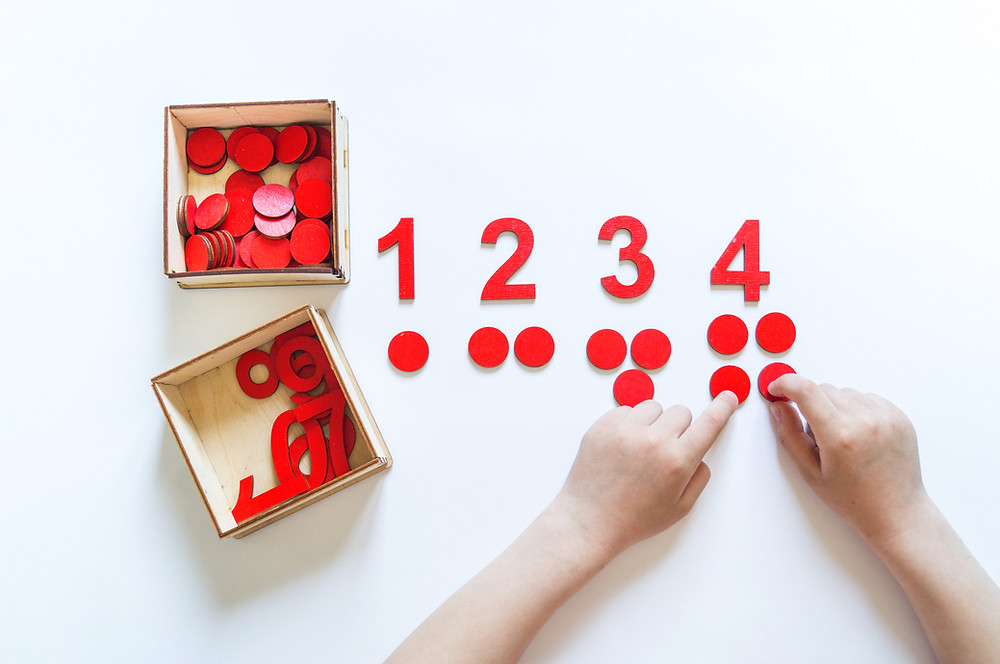Search
• by Blaine Helwig

# Beginning Teachers: When and When Not to Use Tactile Math Manipulatives

As discussed in an earlier blog, “Manipulatives, Pictorial Representation – then, Paper/Pencil,” arithmetic instructional design and student learning should always follow a structured process: tactile/concrete, pictorial representation, paper-pencil. As expected, a newly introduced learning process begins with a hands-on concrete manipulative and transitions to an abstract one.Children need to understand the physical significance of the mathematics – what arithmetic calculations physically represent. For example, the number of fifth graders I have encountered over my public education career that could not draw a simple pictorial representation of a long division problem after correctly computing the quotient of a 3 digit dividend divided by a 1 digit divisor has happened far too often. When this occurs, two things are afoot. Students are not proficient at the mathematics because they do not understand its physical significance, and they have received poorly designed initial core instruction.

Now, do these fifth grade students need a tactile manipulative to learn the physical meaning of division? Possibly - but, probably not a 3 digit dividend divided by a 1 digit divisor. If needed, use small numbers for the dividend and single digit divisors with no remainders. Keep it simple so students are not confused due to large numbers. Then, add the remainders into the process.

So, when do students require a tactile manipulative in elementary school?

This question is valuable for entry-level teachers to fully understand, but the answer depends highly on the instructional situation.

If the mathematical concept being presented is new to students, then a tactile manipulative is always the place to begin the core lessons. For example, in the primary grades, basic addition and subtraction operational facts are new concepts to students. Hence, instruction should begin with a tactile manipulative and transition to a pictorial representation using whole number line modelling and student drawn pictures. Finally, after student understanding, transition to paper-pencil math computations. Revisit the tactile manipulative as needed, but it is highly recommended that students are spirally reviewed in periodic intervals throughout the school year with pictorial representation models for both addition and subtraction to ingrain the physical mathematical meaning of each.If the mathematical situation is not new to students and it is presented as a spiral review, then it is usually not necessary to use the manipulative. The students should be thoroughly reviewed on the pictorial representation. For example, if a third grade teacher introduces basic addition and subtraction concepts previously learned from the primary grades, begin with pictorial representation models (i.e. number line models and whole class tactile object demonstration) and transition to paper-pencil. If a child is receiving special education services, refer to their Individual Education Plan (IEP). It is common that students with learning disabilities require tactile manipulatives.

Simple steps that entry-level teachers may follow to guide their use of manipulatives?

Ask seasoned colleagues for their advice and successful guidance on lesson development. Also, review the major topics in the scope and sequence of grade levels prior to your current teaching assignment – this process will assist in determining the grade level when specific math content was first introduced.

As a principal, I have recommended to novice teachers the following tactile manipulative pedagogical guidance. If students are struggling to understand a concept either pictorially or via paper-pencil computations, it is highly beneficial to reintroduce tactile instruction regardless of the grade level the math concept was first introduced.